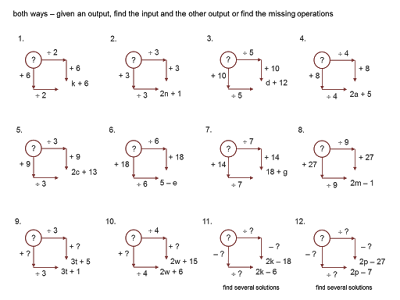median
don steward
mathematics teaching 10 ~ 16

## Thursday, 29 August 2013

### both ways

an important start to algebra work lies in being able to understand the language: the creation, writing and then manipulation of algebraic expressions - deliberately 'playing around' with expressions as a start to algebraic manipulation

prior to work with formulas (including nth term), equations and relationships,
an ability to read an expression as a structured sequence of operations seems an important developmental step - learning the language (conventions) of algebra

this task is a simple enough introduction to expressions, with generalising and proof a central feature

the powerpoint is here
(download it for animations to work)

draw a diagram on the board, without operations

invite students to suggest what they think the lesson is going to be about

then put two operations on the diagram:

these operations are chosen because many students can double a simple number and then add 3

stress that the top operation is the same as the bottom and the right operation the same as the left

ask them again what they think they think they might be asked to do

suggested lesson development:

do you think that when you input a number you will obtain the same answers in these two bottom circles?

don’t leave too much time for them to think…

go through examples for a couple of start numbers (with students contributing)

what seems to be happening?
students will generally notice that the two outputs have a difference of 3.

we seem to have a general rule: it looks as if the two outputs differ by 3
how could we check this rule out?
the focus of the task is on justifying that this difference is always 3

set the task of checking the rule out for themselves: they choose four or five numbers, getting progressively harder if they can (maybe a decimal, maybe a fraction, maybe a negative number, maybe a large number) to test out the rule

go through some of their examples – especially if they think they do not fit the rule
a simple decimal (like 1.2), negative number (- 2) and fraction (3½) can be useful to thoroughly test the rule (and reinforce/develop number skills)

we have properly tested out the rule with many numbers and it does seem to work
how else might we test it out?
students quite often suggest using a very big number

ask them what they think a big number is and keep saying "too small" until you get a million or a billion or a googol etc as a suggestion

how many noughts in a million?
oh, this circle is just too small for me to write all those noughts
I’ll just use ‘m’ for a million if you don’t mind (suggested to me by Gill Hatch)
go across first then down
they usually suggest that a million times 2 can be written as 2m which is neat (the language fitting the way the expression is written conventionally)

adding 3 can be a little more problematic - sometimes someone suggests 2m3
in which case you say that according to the World (convention) 2m3 means 2m × 3
usually they then suggest 2m + 3

going around the other way involves adding 3 first, to get m + 3, then multiplying by 2
usually students will double both terms to obtain 2m + 6

you might also quickly introduce the idea that this can also be written as 2(m + 3)
this is an aside – not dwelt upon, read as 2 lots of m + 3 and parceled using a hand motion

I’d like to choose your own -illions and see if you can repeat what we just did please
students try other ‘-illions’ (b for a billion, d for a dillion, z for a zillion, t for a thousand, g for a googol etc.)

they could also, later, start with an input expression: 2d + 5, or 3n - 1 etc., still checking that the difference is always 3

Anya, can you think of a number and don’t tell us what it is please?
what shall I write for Anya’s number?
and what does this 'a' stand for? (Anya’s number rather than Anya)

what do I write here?
and here? etc.
now the letter is being used as a variable - albeit one that Anya knows the value of...

ok, so what will the difference be at the end?
after going through the steps - but we don’t know what Anya’s number was, so this must prove that the result will always be 3

some might devise or understand a diagrammatic view:

you could suggest using 'p' for any old plank length (that we don't know and aren't interested in)

but we are interested in Anya's number
can you tell us what the two outputs were?
so how can we work out what Anya’s number was?

either worked out by going backwards, or trial and improvement

later work can involve starting with an expression and transforming it:

the operations (+ 3) then (x 2) can be varied and further general rules sought - building to an overall rule for any 'multiply by' number and any 'add on' numberas an extension, find the input expression from one output